# Countable-dimensional real projective space

Jump to: navigation, search
This article is about a particular topological space (uniquely determined up to homeomorphism)|View a complete list of particular topological spaces

## Definition

### As a projective space

This topological space is the projective space corresponding to a countable-dimensional vector space over the real numbers. Explicitly, denote by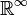$\R^\infty$ the space of all sequences of real numbers with at most finitely many nonzero entries, where the addition and scalar multiplication are coordinate-wise. This is a countable-dimensional vector space over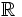$\R$. We thus have an action of the multiplicative group$\R^*$ on it by scalar multiplication. The quotient of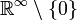$\R^\infty \setminus \{ 0 \}$ by this action, given the quotient topology, is defined as the countable-dimensional real projective space and is denoted as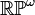$\R\mathbb{P}^\omega$ or$\R\mathbb{P}^\infty$.

### As a quotient of a countable-dimensional sphere by antipode identification

Define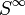$S^\infty$, the countable-dimensional sphere, as follows: denote by$\R^\infty$ the space of all sequences of real numbers with at most finitely many nonzero entries, where the addition and scalar multiplication are coordinate-wise.$S^\infty$ is the subset of$\R^\infty$ comprising those sequences whose sum of squares of entries is$1$.$\R\mathbb{P}^\infty$ is defined as the quotient of$S^\infty$ by the following equivalence relation: any element is identified with its antipode, i.e., the element obtained by taking negatives of all the entries. This quotient is equipped with the quotient topology.

## As a classifying space

Further information: Groupprops:Group cohomology of cyclic group:Z2 (on the Group Properties Wiki)

This topological space is a path-connected aspherical space and its fundamental group is cyclic of order two. This follows from the definition in terms of the countable-dimensional sphere (making the countable-dimensional sphere its double cover) and the fact that the countable-dimensional sphere is contractible

Thus, it can be viewed as a classifying space for the cyclic group of order two. In particular, this means that the topological homology and cohomology groups of this space are the same as the group homology and cohomology groups of cyclic group:Z2.

## Algebraic topology

### Homology

Further information: homology of countable-dimensional real projective space

### Cohomology

Further information: cohomology of countable-dimensional real projective space

### Homotopy

The zeroth homotopy is a one-point set, the first homotopy group is cyclic of order two, and all higher homotopy groups are zero (i.e., the space is an aspherical space). This follows because the universal cover, the countable-dimensional sphere, is a double cover and also the fact that countable-dimensional sphere is contractible.